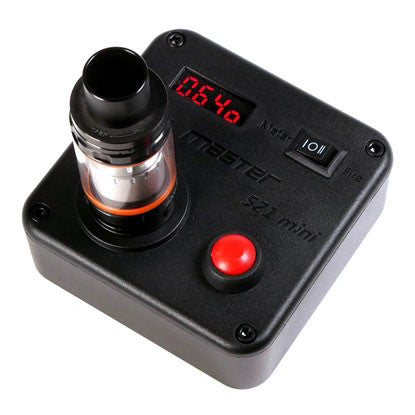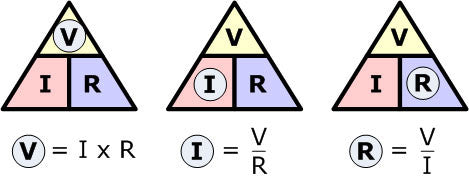# Safe Vaping With Ohm's Law

Safety in vaping needs a good understanding with battery safety and knowing your battery limitations. Then, you must consider to have a knowledge about Ohm's Law in order for you to tweak your coil builds and look for your vaping "Sweet Spot".

First, what is Ohm's Law? Ohm's Law is the mathematical relationship among electric current, resistance, and voltage. I can hear your mind say "Really? Am I going to study it?". My answer to that is yes, but you don't really have to compute manually because there are a lot of ohm's law calculators online. Also, it will be really helpful if you have your own Atomizer Ohm Checker like this.If you have those, it will be easy for you to experiment your build and check if it is within the capacity of your battery. But how would you know if it is safe without understanding the ohm's law itself? So here we go. Let us start.

There's nothing special in Ohm's Law. It is just a few formulas, and anyone can definitely learn and solve the formulas with using any calculators at home.V = Voltage (The Battery)

I  = Current (The Coil Amperage)

It is simple! If you want to know the current drawn to a resistance of your coil simply look at the triangle and you will notice that  the V(Voltage) is above R(Resistance). So it says I is equal to V over R or I is equal to V(Voltage) divided by R(Resistance).

For example:

I = 3.7V ÷ 0.5Ω

I = 7.4A(Amperes)

And so if you want to know the P(power) generated at the coil or what we call Wattage, simply solve for V(Voltage).

That is V(Voltage) is equal to I(Ampere) multiplied to the R(Resistance of the coil)

For example:

P = 3.7V x 7.4A

P = 27.38W(Wattage)

Lastly, calculating the R(Resistance). This is the most important part of Ohm's Law in Vaping specially if you are using a mechanical mod. You probably want to determine the lowest coil resistance that you can safely use the battery without exceeding the CDR of the battery.

If you would look at the triangle again, you can see that R is in the lower right part and that is the missing value. So looking at the triangle again, V(Voltage) is above the I(Ampere). So it says R(Resistance) is equal to V(Voltage) over I(Ampere) or R(Resistance) is equal to V(Voltage) divided by I(Ampere).

For example:

The CDR of the battery is 20A, you might want to target 19A or below.

Also we already know that the max voltage of a battery is 4.2V

So it goes like this:

R = 4.2V ÷ 19A

R = 0.22Ω

So it says that  anything lower than 0.22Ω will exceed the CDR of your battery and you will have a big chance that your battery will vent out or explode.

Also, I have an important advice for you guys. This is from my mentor and it became very useful for me to explore and make different kinds of builds just to obtain my "Sweet Spot" in Vaping. In calculating using ohm's law, ALWAYS assume that the voltage of your battery is equal to a fully charged battery which is 4.2V for a single battery mod or parallel configuration mechanical mod and 8.4V for a dual battery series configuration mod.